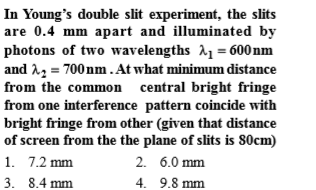A parallel beam of moving electrons is incident normal on a narrow slit. A fluorescent screen is placed at a large distance from the slit. If the slit is further narrowed, then which of the following statement is correct

1.  The diffraction pattern is not observed on the screen in case of electrons

2.  The angular width of the central maximum of the diffraction pattern will increase

3.  The angular width of central maximum will decrease

4.  angular with of central maximum will remain same

Complete Question Bank + Test Series
Complete Question Bank

Difficulty Level:Complete Question Bank + Test Series
Complete Question Bank

Difficulty Level:

A diffraction pattern is observed using a beam of red light. What will happen if the red light is replaced by blue light?

1.  No change takes place

2.  Diffraction bands become narrower

4.  Diffraction pattern disappears

Complete Question Bank + Test Series
Complete Question Bank

Difficulty Level:

Phenomen of polarisation justify the nature of light

1.  longitudinal

2.  transverse

3.  both

4.  geometrical

Complete Question Bank + Test Series
Complete Question Bank

Difficulty Level:

Two waves of intensity ratio 9:1 interfere to produce fringes in a young's double-slit experiment, the ratio of  intensity at maxima  to the intensity at minima is

1.  4:1

2.  9:1

3.  81:1

4.  9:4

Complete Question Bank + Test Series
Complete Question Bank

Difficulty Level:

Interference fringes produced using white light in a double-slit arrangement. When a mica sheet of uniform thickness of the refractive index 1.6 (relative to air) is placed in the path of light from one of the slits, the central fringe moves by a distance. This distance is equal to the width of 30 interference bands if the light of wavelength 4800 $\stackrel{\mathrm{o}}{\mathrm{A}}$ is used The thickness (in $\mu$m) of mica is —

1.  90

2.  12

3.  14

4.  24

Complete Question Bank + Test Series
Complete Question Bank

Difficulty Level:

Young's double slit experiment is first performed in air and then in a medium other than air. It is found that 8th bright fringe in the medium lies where 5th dark fringe lies in air. The refractive index of the medium is nearly

(a)1.25

(b) 1.59

(c) 1.69

(d) 1.78

Complete Question Bank + Test Series
Complete Question Bank

Difficulty Level:

Two polarids  are placed with their axis perpendicular to each other. Unpolarised light ${I}_{o}$ is incident on ${P}_{1}$. A third polaroid ${P}_{3}$ is kept in between  such that its axis makes an angle ${45}^{°}$ with that of ${P}_{1}$. The intensity of transmitted light through ${P}_{2}$

(a)$\frac{{I}_{o}}{2}$

(b) $\frac{{I}_{o}}{4}$

(c) $\frac{{I}_{o}}{8}$

(d) $\frac{{I}_{o}}{16}$

Complete Question Bank + Test Series
Complete Question Bank

Difficulty Level:

The interference pattern is obtained with two coherent light sources of intensity ratio n. In the interference pattern, the ratio $\frac{{I}_{max}-{I}_{min}}{{I}_{max}+{I}_{min}}$ will be

(a) $\frac{\sqrt{n}}{n+1}$             (b) $\frac{2\sqrt{n}}{n+1}$

(c) $\frac{\sqrt{n}}{{\left(n+1\right)}^{2}}$         (d) $\frac{2\sqrt{n}}{{\left(n+1\right)}^{2}}$

Complete Question Bank + Test Series
Complete Question Bank

Difficulty Level:

A linear aperture whose width is 0.02 cm is placed immediately in front of a lens of focal length 60 cm. The aperture is illuminated normally by a parallel beam of wavelength $5×{10}^{-5}$ cm. The distance of the first dark band of the diffraction pattern from the centre of the screen is-

(a) 0.10 cm             (b) 0.25 cm

(c) 0.20 cm             (d) 0.15 cm